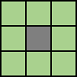# bwconvhull

Generate convex hull image from binary image

## Syntax

``CH = bwconvhull(BW)``
``CH = bwconvhull(BW,method)``
``CH = bwconvhull(BW,'objects',conn)``

## Description

example

````CH = bwconvhull(BW)` computes the convex hull of all objects in `BW` and returns `CH`, a binary convex hull image.```
````CH = bwconvhull(BW,method)` specifies the desired method for computing the convex hull image.```
````CH = bwconvhull(BW,'objects',conn)` specifies the desired connectivity used when defining individual foreground objects.```

## Examples

collapse all

Read a grayscale image into the workspace. Convert it into a binary image and calculate the union binary convex hull. Finally, calculate the objects convex hull and display all the images in one figure window.

```subplot(2,2,1); I = imread('coins.png'); imshow(I); title('Original'); subplot(2,2,2); BW = I > 100; imshow(BW); title('Binary'); subplot(2,2,3); CH = bwconvhull(BW); imshow(CH); title('Union Convex Hull'); subplot(2,2,4); CH_objects = bwconvhull(BW,'objects'); imshow(CH_objects); title('Objects Convex Hull');```## Input Arguments

collapse all

Input binary image, specified as a 2-D logical matrix.

Data Types: `single` | `double` | `int8` | `int16` | `int32` | `int64` | `uint8` | `uint16` | `uint32` | `uint64` | `logical`

Method used to compute the convex hull, specified as one of the following:

ValueDescription
`'union'`Compute the convex hull of all foreground objects, treating them as a single object
`'objects'`Compute the convex hull of each connected component of `BW` individually. `CH` contains the convex hulls of each connected component.

Data Types: `char` | `string`

Pixel connectivity, specified as one of these values The `conn` parameter is only valid when the `method` is `'objects'`.

Value

Meaning

Two-Dimensional Connectivities

`4`

Pixels are connected if their edges touch. Two adjoining pixels are part of the same object if they are both on and are connected along the horizontal or vertical direction.Current pixel is shown in gray.

`8`

Pixels are connected if their edges or corners touch. Two adjoining pixels are part of the same object if they are both on and are connected along the horizontal, vertical, or diagonal direction.Current pixel is shown in gray.

Connectivity can also be defined in a more general way by specifying a 3-by-3 matrix of `0`s and `1`s. The `1`-valued elements define neighborhood locations relative to the center element of `conn`. The matrix must be symmetric about its center element.

Data Types: `double`

## Output Arguments

collapse all

Binary mask of the convex hull of all foreground objects in the input image, returned as a 2-D logical matrix.

## Version History

Introduced in R2011a